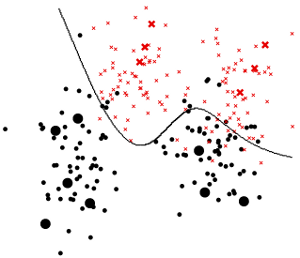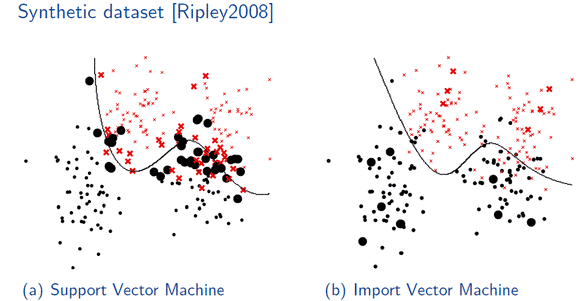# Import Vector Machine Classifier

The Import Vector Machines (Zhu and Hastie 2005) are a sparse, discriminative and probabilistic classifier.

The algorithm is based on the Kernel Logistic Regression model, but uses only a few data points to define the decision hyperplane in the feature space. These data points are called import vectors.

The Import Vector Machine shows similar results to the widely used Support Vector Machine, but has a probabilistic output.

.

.

## Publications

The Import Vector Machine is described in this Journal article, on which our implementation is based on:

Ji Zhu and Trevor Hastie, “Kernel Logistic Regression and the Import Vector Machine“, Journal of Computational and Graphical Statistics, 2005, Vol. 14(1), pp. 185-205.(download)

Our Import Vector Machine algorithm can be found in:

Ribana Roscher and Wolfgang Förstner and Björn Waske, “I2VM: Incremental import vector machines”, Image and Vision Computing, May, 2012. Vol. 30(4-5), pp. 263-278.

Please cite the source code if you are using it in your work.

.

## Sample Result

Ripley dataset• classification result with rbf-kernel and empirically determined kernel and regularization parameter
• bold points are the import vectors

## Comparison with Other Algorithms[SVM] Chang, C.-C. and Lin, C.-J. LIBSVM : a library for support vector machines.